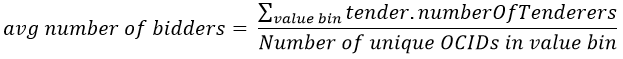# Average number of bidders for tenders, disaggregated by contract value

This report depicts the average number of bidders for tenders, disaggregated by the contract value. The contract value is separated into pre-defined ranges:

• up to 10.000 ZMW
• 10.001 – 500.000 ZMW
• 500.001 – 5.000.000 ZMW
• 5.000.001 – 50.000.000 ZMW
• more than 50.000.000 ZMW

The average number of bidders is calculated as follows:In other words, the average number of tenderers is calculated over all contracts whose value falls within a corresponding bin. As an example, to calculate the average number of tenderers for the first bin of “less than 10.000 ZMW”, all contracts whose value is less than or equal to 10.000 ZMW are selected. The average number of tenderers is calculated for the specific bin by summing the individual values for each contract and dividing by the number of contracts in the bin.

The data is published according to the schema defined by the Open Contracting Data Standard (OCDS).

Please note that all dates/times are stored in UTC. Please use the appropriate tools to convert these to your local timezone if you export the chart data.